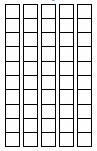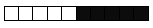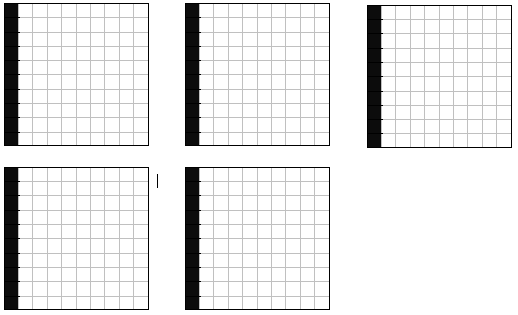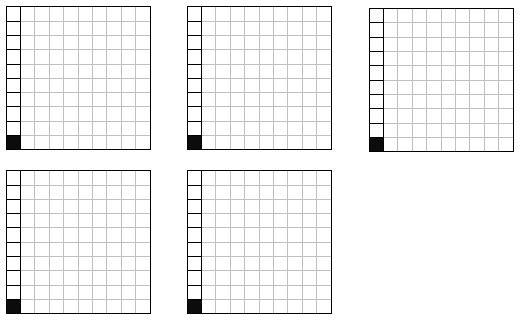# Base-Ten Block Multiplication

Three students have used base-10 blocks to provide an explanation of .01 x .5. Evaluate each of their explanations – and explain what you believe is each student’s mathematical thinking in his/her particular explanation and how they use the blocks to arrive at their solution. If their answer is incorrect, explain how you would address their misconception(s).

Sheila
If one long is .1 then 5 longs is .5 and one cube is .01. Since there are 50 cubes in 5 longs the answer is 50.Nacole
I agree with Sheila that we should use longs to figure this out but I don’t agree with her answer. If I let a long equal .01 then five-tenths of a long is 5 cubes. Therefore a cube is .001 so the answer is .005.Mark
I let the flat be .1 so one long is .01 and five flats is .5. There are five longs so the answer is .05.Jerome
I like how Mark used the flats but I disagree with his answer. If one long is .01 then one cube is .001 so five of them is .005.Adapted from: Fostering Children’s Mathematical Power: An Investigative Approach To K-8 Mathematics Instruction by Arthur J. Baroody and Ronald T. Coslick, Lawrence Erlbaum Associates, 1998.